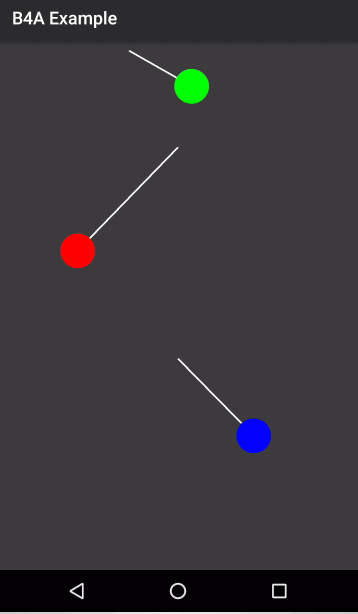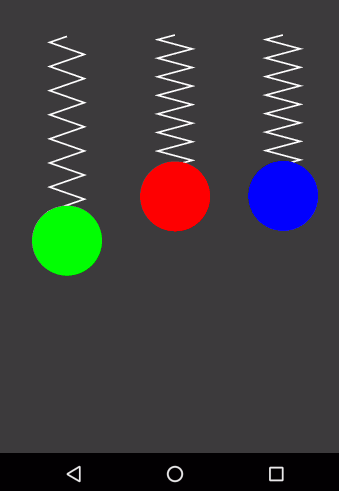# Android Code SnippetMathemetics Pendulum

#### Erel

Staff member
A not very useful animated pendulum.(it is smoother on a real device in release mode)

B4X:
``````Sub Activity_Create(FirstTime As Boolean)
Dim cvs As Canvas
cvs.Initialize(Activity)
DrawPendulum (cvs, 50%x, 20%y, 40%x, -45, Colors.Red)
DrawPendulum (cvs, 50%x, 60%y, 30%x, 45, Colors.Blue)
DrawPendulum (cvs, 150dip, 10dip, 20%x, 70, Colors.Green)
End Sub

Sub DrawPendulum (cvs As Canvas, cx As Float, cy As Float, l As Float, ZeroAngle As Float, clr As Int)
Dim teta0 As Float = ZeroAngle / 180 * cPI
Dim start As Long = DateTime.Now
Dim w As Float = Sqrt(1000 / l) / 1000
Dim x2, y2 As Float
Do While True
Sleep(10)
Dim t As Int = DateTime.Now - start
Dim teta As Float = teta0 * Cos(w * t)
cvs.DrawLine(cx, cy, x2, y2, Colors.Transparent, 2dip)
cvs.DrawCircle(x2, y2, 20dip, Colors.Transparent, True, 1dip)
x2 = cx + l * Sin(teta)
y2 = cy + l * Cos(teta)
cvs.DrawLine(cx, cy, x2, y2, Colors.White, 2dip)
cvs.DrawCircle(x2, y2, 20dip, clr, True, 1dip)
Activity.Invalidate
Loop
End Sub``````

#### GMan

##### Well-Known Member
But nice to look @#### udg

##### Expert
Command me, master... (I'm hypnothized by the green one..eheh)

•GMan, Erel and DonManfred

#### Javier Donayre

##### Member
Next one, the "inverted" animated pendulum!!#### Erel

Staff member
I'm afraid that unstable systems are above my physics level...B4X:
``````Sub Activity_Create(FirstTime As Boolean)
Dim cvs As Canvas
cvs.Initialize(Activity)
DrawSpring(cvs, 50%x, 20%y, 40%y, 0.004, Colors.Red)
DrawSpring(cvs, 20%x, 20%y, 40%y, 0.002, Colors.Green)
DrawSpring(cvs, 80%x, 20%y, 40%y, 0.003, Colors.Blue)
End Sub

Sub DrawSpring(cvs As Canvas, cx As Float, cy As Float, l As Float, w As Float, clr As Int)
Dim start As Long = DateTime.Now
Dim x As Float
Dim num As Int = 8
Dim width As Int = 20dip
Dim p As Path
Do While True
Sleep(10)
If p.IsInitialized Then
cvs.DrawPath(p, Colors.Transparent, False, 2dip)
cvs.DrawCircle(cx, cy + l + x, 40dip, Colors.Transparent, True, 1dip)
End If
Dim t As Int = DateTime.Now - start
x = 60dip * Cos(w * t - 0.5)
Dim h As Float = (l + x - 20dip) / num
p.Initialize(cx, cy + h/4)
For i = 0 To num - 1
p.LineTo(cx - width, cy + h / 2 + h * i)
p.LineTo(cx + width, cy + h + h * i)
Next
cvs.DrawPath(p, Colors.White, False, 2dip)
cvs.DrawCircle(cx, cy + l + x, 40dip, clr, True, 1dip)
Activity.Invalidate
Loop
End Sub``````